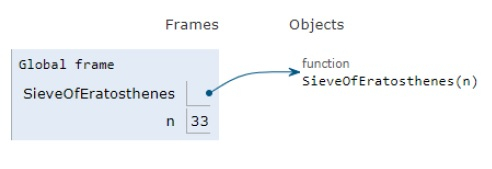# Python Program for Sieve of Eratosthenes

Problem statement − We are given a number n, we need to print all primes smaller than or equal to n. Constraint: n is a small number.

Now let’s observe the solution in the implementation below −

## Example

def SieveOfEratosthenes(n):
# array of type boolean with True values in it
prime = [True for i in range(n + 1)]
p = 2
while (p * p <= n):
# If it remain unchanged it is prime
if (prime[p] == True):
# updating all the multiples
for i in range(p * 2, n + 1, p):
prime[i] = False
p += 1
prime= False
prime= False
# Print
for p in range(n + 1):
if prime[p]:
print (p,end=" ")
# main
if __name__=='__main__':
n = 33
print ("The prime numbers smaller than or equal to", n,"is")
SieveOfEratosthenes(n)

## Output

The prime numbers smaller than or equal to 33 is
2 3 5 7 11 13 17 19 23 29 31All the variables are declared in the local scope and their references are seen in the figure above.

## Conclusion

In this article, we have learned about how we can make a Python Program for Sieve of Eratosthenes